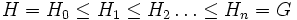# Almost subnormal subgroup

This article defines a subgroup property: a property that can be evaluated to true/false given a group and a subgroup thereof, invariant under subgroup equivalence. View a complete list of subgroup properties[SHOW MORE]
This is a variation of subnormal subgroup|Find other variations of subnormal subgroup |

## Definition

A subgroup$H$ of a group$G$ is termed an almost subnormal subgroup (or sometimes f-subnormal subgroup) of$G$ if there exists a chain$H = H_0 \le H_1 \le H_2 \dots \le H_n = G$, such that for$0 \le i \le n - 1$,$H_i$ is either a normal subgroup or a subgroup of finite index in$H_{i + 1}$.

## Relation with other properties

### Stronger properties

Property Meaning Proof of implication Proof of strictness (reverse implication failure) Intermediate notions
Normal subgroup Almost normal subgroup, Join-transitively almost subnormal subgroup, Nearly normal subgroup, Subnormal subgroup|FULL LIST, MORE INFO
Subnormal subgroup chain from subgroup to group, each normal in successor |FULL LIST, MORE INFO
Subgroup of finite index has finite index in the whole group Almost normal subgroup, Join-transitively almost subnormal subgroup, Nearly normal subgroup|FULL LIST, MORE INFO
Almost normal subgroup has finitely many conjugate subgroups |FULL LIST, MORE INFO
Nearly normal subgroup has finite index in its normal closure |FULL LIST, MORE INFO